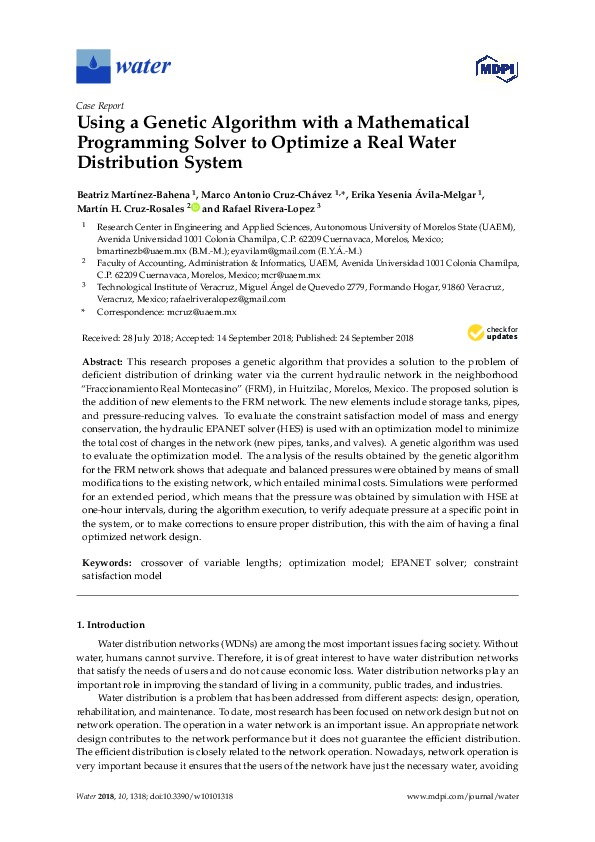# Using a GA with a Mathematical Programming Solver to Optimize a Real Water Distribution SystemUsing a Genetic Algorithm with a Mathematical Programming Solver to Optimize a Real Water Distribution System

Beatriz Martínez-Bahena, Marco Antonio Cruz-Chávez, Erika Yesenia Ávila-Melgar, Martín H. Cruz-Rosales and Rafael Rivera-Lopez

Abstract

This research proposes a genetic algorithm that provides a solution to the problem of deficient distribution of drinking water via the current hydraulic network in the neighborhood “Fraccionamiento Real Montecasino” (FRM), in Huitzilac, Morelos, Mexico. The proposed solution is the addition of new elements to the FRM network. The new elements include storage tanks, pipes, and pressure-reducing valves.

To evaluate the constraint satisfaction model of mass and energy conservation, the hydraulic EPANET solver (HES) is used with an optimization model to minimize the total cost of changes in the network (new pipes, tanks, and valves). A genetic algorithm was used to evaluate the optimization model. The analysis of the results obtained by the genetic algorithm for the FRM network shows that adequate and balanced pressures were obtained by means of small modifications to the existing network, which entailed minimal costs.

Simulations were performed for an extended period, which means that the pressure was obtained by simulation with HSE at one-hour intervals, during the algorithm execution, to verify adequate pressure at a specific point in the system, or to make corrections to ensure proper distribution, this with the aim of having a final optimized network design.

Keywords : crossover of variable lengths; optimization model; EPANET solver; constraint satisfaction model

Water 2018, 10(10), 1318; https://doi.org/10.3390/w10101318

Martínez-Bahena, B.; Cruz-Chávez, M.A.; Ávila-Melgar, E.Y.; Cruz-Rosales, M.H.; Rivera-Lopez, R. Using a Genetic Algorithm with a Mathematical Programming Solver to Optimize a Real Water Distribution System.  Water   2018 10 , 1318.

Source: MDPI